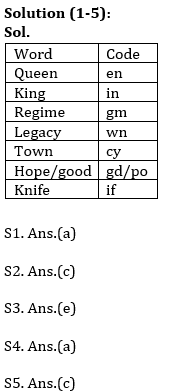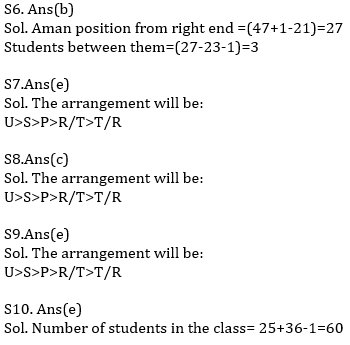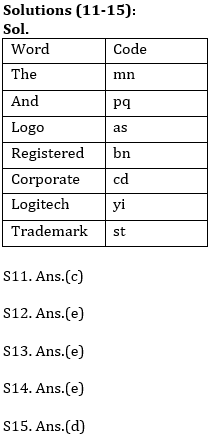Latest Banking jobs   »

# Reasoning Ability Quiz For LIC AAO 2023- 18th January

Directions (1-5): Study the following information carefully and answer the questions given below:
In a certain code language:
‘Queen King Regime’ is written as ‘en in gm’,
‘Regime legacy town’ is written as ‘gm cy wn’,
‘Hope town good’ is written as ‘cy gd po’
‘Regime King Knife’ is written as ‘in gm if’.

Q1. What is the code for ‘legacy knife’ in the given code language?
(a) wn if
(b) gm wn
(c) gd wn
(d) if cy
(e) None of these

Q2. What is the code for ‘Regime’ in the given code language?
(a) en
(b) in
(c) gm
(d) if
(e) None of these

Q3. What is the code for ‘Good’ in the given code language?
(a) gd
(b) po
(c) cy
(d) if
(e) Can’t be determined

Q4. What may be the code for ‘King Hope’ in the given code language?
(a) in gd
(b) if po
(c) in cy
(d) Either (a) or (c)
(e) None of these

Q5. What is the code for ‘Town’?
(a) gd
(b) po
(c) cy
(d) in
(e) None of these

Q6. Aman is 21st from the left end of a row of 47 students and Monika is 23rd from the right end in the same row. How many students are there between them in the row?
(a) 1
(b) 3
(c) 2
(d) 5
(e) None of these

Directions (7-9): Study the following information carefully and answer the given questions.
Amongst five persons, P, R, S, T and U, each has different weight. P’s weight is more than R and T. S weight is less than U. Only two persons weight is more than P, whose weight is 65kg. Youngest person weight is 55kg.

Q7. Who among the following is youngest person?
(a) U
(b) R
(c) S
(d) T
(e) Can’t be determined

Q8. If U’s weight is 70kg, then what may be the weight of S?
(a) 63
(b) 64
(c) 68
(d) 72
(e) None of these

Q9. How many persons are heavier than R?
(a) One
(b) Two
(c) Three
(d) None
(e) Can’t be determined

Q10. Adarsh ranked 25th from the top and 36th from the bottom in a class. How many students are there in the class?
(a) 61
(b) 59
(c) 52
(d) 58
(e) 60

Directions (11-15): Study the following information carefully and answer the given questions.
In a certain code language,
‘Logitech And The Logo’ is coded as ‘yi pq mn as’
‘Registered Trademark And The’ is coded as ‘mn bn st pq ‘
‘Registered Corporate The Logo’ is coded as ‘cd as mn bn’

Q11. Which of the following is the code for ‘and’?
(a) st
(b) bn
(c) pq
(d) mn
(e) None of these

Q12. Which of the following word is coded as ‘mn’?
(a) Corporate
(b) Logo
(d) Registered
(e) None of these

Q13. What may be the code of ‘Corporate society’?
(a) st pq
(b) cd mn
(c) pq bn
(d) mn pq
(e) cd qw

Q14. Which of the following code is coded for ‘logitech’?
(a) mn
(b) pq
(c) bn
(d) cd
(e) None of these

Q15. What will be the code of ‘the Logo’?
(a) st pq
(b) cd as
(c) cd pq
(d) mn as
(e) st bn

Solutions## FAQs

### When will the LIC AAO Prelims exam be held?

The LIC AAO Prelims exam will be held on 17th & 20th of February 2023 (tentatively).

#### Congratulations!